﻿ The Chaos Game and the Sierpinski Fraction

# The Chaos Game and the Sierpinski fraction

The chaos game is an algorithm designed to draw certain fractals which was first proposed by Michael Barnsley in his book Fractals Everywhere. [Note: The version of the chaos game introduced here is slightly more general than the original version by Barnsley, but the general idea is the same] First pick a set of $$n$$ vertices and a fraction $$r$$. Then, draw a point at random and iterate over the following steps:

1. Randomly choose one of the vertices of the set.
2. Draw a point at a fraction $$r$$ of the distance between the vertex chosen in the previous step and the last point drawn.

The result of this process is sometimes a fractal. Figure 1 shows the result of the chaos game after 50,000 iterations with the vertices of an equilateral triangle as the set of vertices ($$n=3$$ and $$r=\frac{1}{2}$$).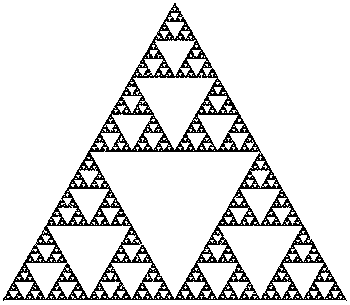Figure 1. Chaos game in a regular polygon with $$n=3$$ and $$r=\frac{1}{2}$$ after 50,000 iterations.

In this particular case the result of the chaos game is indeed a fractal. We will use this fractal (known as the Sierpinski Triangle) as a starting point in our study of the chaos game.

### The Sierpinski Triangle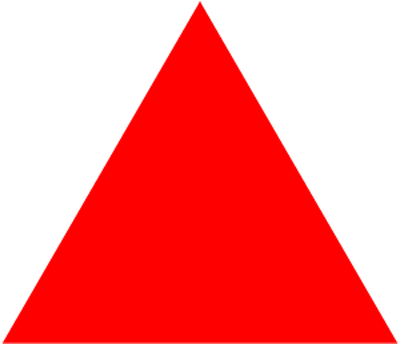Figure 2. Sierpinski triangle generated using Trema removal. Click to enlarge.

To understand exactly why the Sierpinski triangle is the result of the chaos game depicted in Figure 1 we first need to understand a few facts about the Sierpinski triangle itself. There are many different ways to precisely define what the Sierpinski triangle is, however the most intuitive way to do it is probably using trema removal (pictured in Figure 2). First, start with a full triangle (not necesarily equilateral), then remove the triangle formed by joining the midpoints of each of the sides of the original triangle (the removed triangle is called a trema). Then repeat this process with each of the three remaining triangles. The result after repeating this process an infinite number of times is the Sierpinksi triangle. Notice that in each step the triangles left after the trema is removed are half the size of the original triangle. Also notice that the only points that are never part of a trema are those that at some point in the process are the vertices of one of the triangles, thus, the Sierpinski triangle is precisely the set of vertices of all the triangles involved in the trema removal. An interesting fact about the Sierpinski triangle that is not directly related to our study is that its area is zero but its perimeter diverges. If you're interested you can click below to view the proofs for these facts.

Show proofs.

Let $$A$$ be the area of the triangle before any tremas have been removed. After drawing the lines between the midpoints of the sides we get four new triangles. The length of the sides of the new triangles is half the length of the sides of the original triangle, so the area of each one of these new triangles is $$\frac{A}{4}$$. Since the first trema is one of these triangles, then the area left after removing the first trema is $$A - \frac{A}{4}$$. Next, a new trema is removed from each of the remaning triangles. Since the area of each one of these triangles is $$\frac{A}{4}$$ and we have shown that the tremas have one fourth of the area of the triangle from where they come from, then the area of the new tremas is $$\frac{A}{16}$$. This time however, three tremas where removed instead of just one so the area of what is left is $$A - \frac{A}{4} - \frac{3A}{16}$$. Since each triangle is divided into three smaller triangles, then in each step there are three times more triangles than in the previous step. Thus, after $$n$$ steps, the area of what is left is $$A - \frac{1}{4}A - \frac{3}{16}A - \cdots - \frac{3^{n-1}}{4^n}$$ Factoring $$A$$ in the previous expression we get: $$A \left[1 - \left(\frac{1}{4} + \frac{3}{16} + \cdots + \frac{3^{n-1}}{4^n}\right)\right]$$ Since the Sierpinski triangle is the result of of an infinite number of trema removals then the area of the Sierpinski triangle is: \begin{align} \lim_{n \to \infty} A \left[1 - \left(\frac{1}{4} + \frac{3}{16} + \cdots + \frac{3^{n-1}}{4^n}\right)\right] &= \lim_{n \to \infty} A \left[1 - \sum_{k=1}^n \frac{3^{k-1}}{4^k} \right] \\ &= A \left[1 - \lim_{n \to \infty} \sum_{k=1}^n \frac{3^{k-1}}{4^k} \right] = A \left[1 - \lim_{n \to \infty} \sum_{k=0}^n \frac{3^k}{4^{k+1}} \right] = A \left[1 - \lim_{n \to \infty} \sum_{k=0}^n {\frac{1}{4}\left(\frac{3}{4}\right)^k} \right] \end{align} Now we notice that the limit in the above expression is a geometric series whose first term is $$\frac{1}{4}$$ and ratio is $$\frac{3}{4}$$, and since $$\left|\frac{3}{4}\right| < 1$$, then the series converges and $$\sum_{k=0}^\infty \left[ \frac{1}{4} \cdot \left( \frac{3}{4} \right)^k \right] = \frac{\frac{1}{4}}{1-\frac{3}{4}} = \frac{\frac{1}{4}}{\frac{1}{4}} = 1$$ Substituting in the espression above we get that the are of the Sierpinski triangle is $$A(1-1) = 0$$ ■

Now let's consider the perimeter. Let $$P$$ be the perimeter of the original triangle. Since the triangles left after a trema is removed are congruent to one another and each of the sides of the original triangle was divided by its midpoint, then after the first trema removal there are three triangles left, each with a perimeter of $$\frac{P}{2}$$. Then, the perimeter of all of the triangles put together is $$3\frac{L}{2}$$. As above, since there are three times more triangles after each step and the perimeter of each triangle is half the perimeter of the triangles from the previous step, then perimeter after $$n$$ steps is given by $$\left( \frac{3}{2} \right)^n$$. Thus, the perimeter of the Siperpinski triangle is $$\lim_{n \to \infty} \frac{3^n}{2^n}$$ But since the numerator of the fraction will always be bigger than the denominator the this limit diverges. Therefore as the number of steps goes to infinity so does the perimeter. ■

### The Chaos Game Explained

It is now time to delve into what makes the chaogs game work. The chaos games is a particular case of a more general concept called an iterated function system.. Formally, an iterated function system (IFS for short) is a finite set of contraction mappings on a complete metric space, that is, if $$(X,d)$$ is a metric space, then $$\{F_i : X \to X \; | \; 1 \leq i \leq n \}, \; n \in \mathbb N$$ is an IFS if all the $$F_i$$ are contractions. Don't worry if you don't understand what that means though, you don't really need to. Suffice it to say that an IFS is a set of mappings from one space onto itself with the property that the distance between any two points in the space gets smaller after the mapping is applied. For the time being we will only consider the following IFS: \begin{align} F_A(x,y) &= \left(\frac{x}{2},\frac{y}{2}\right) \\ F_B(x,y) &= \left(\frac{x}{2} + \frac{1}{2},\frac{y}{2} + \frac{1}{2}\right) \\ F_C(x,y) &= \left(\frac{x}{2} + \frac{1}{2},\frac{y}{2}\right) \end{align} where $$F_i : \mathbb R^2 \to \mathbb R^2$$ for $$i \in \{A,B,C\}$$. We won't go into the details of why this is an IFS here, but it should be fairly straightforward for anyone who understdood the definition. Instead we will take a look at what this IFS does gemetrically. Consider the triangle formed by the points $$A,B,C \in \mathbb R^2$$ where $$A = (0,0)$$, $$B = (1,1)$$ and $$C = (1,0)$$. This triangle is shown in Figure 3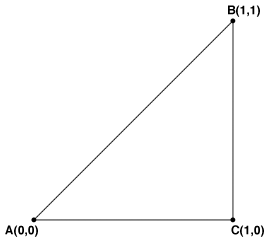Figure 3. Illustration of the IFS. Show mappings: $$F_A$$, $$F_B$$, and $$F_C$$.

Pressing the $$F_A$$,$$F_B$$ and $$F_C$$ labels in Figure 3 will toggle the visibility of the triangles formed by applying the specified mapping to points $$A$$, $$B$$ and $$C$$. As you can see, all three transofmrations map the triangle $$ABC$$ to a triangle half the size (that's what $$\frac{x}{2}$$ and $$\frac{y}{2}$$ do in the definition of the mappings) and then translates the shrinked triangle so that the point that remains fixed is either $$A$$, $$B$$ or $$C$$ (that's what the "$$+\frac{1}{2}$$"s do).

Now consider what would happen if we applied each mapping not only to points $$A$$, $$B$$, and $$C$$, but also to the points we got by applying the mappings the first time. The result is shown in Figure 4, remember that the lines are only there to make things easier to understand, but are not part of the IFS mappings.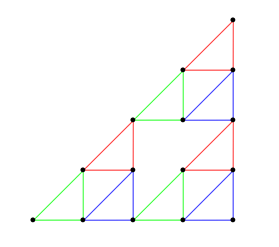Figure 4.

Essentially, both the vertices of the triangles and the midpoints of the triangle get shrkinked into the smaller triangle and are then translated. By now you should have noticed the resemblance to the trema removals. Remember that after an infinite amount of tremas are removed the only points left are those that were vertices of a triangle left at some step. On the other hand, if we start with just the points $$A$$, $$B$$, and $$C$$ and apply all of our mappings then what we get is exactly the set of points from the Sierpinski triangle that are from the triangles that show up after the first trema removal. Similarly, if we now apply all three of our mappings to all of these points then what we get are the points in the Sierpinski triangle that show up after the second trema removal. Thus, if we start with just the points $$A$$, $$B$$, and $$C$$ and keep applying all three of our transformations recursively then what we get after an infinite number of interations is precisely the Sierpinksi triangle.

Now let's focus on $$F_C$$ for a moment and consider a point $$(x,y) \in \mathbb R^2$$. Then, the midpoint between $$(x,y)$$ and $$C$$ is given by $$\left(\frac{x+1}{2},\frac{y+0}{2}\right) = \left(\frac{x}{2} + \frac{1}{2},\frac{y}{2}\right)$$ which is exactly $$F_C$$. Similarly $$F_A$$ and $$F_B$$ are the functions that map every point to its midpoint with respecto to $$A$$ or $$B$$. So as it turns out, these transformations are exactly the same as the transformations used in the chaos game with $$A$$, $$B$$, and $$C$$ as the initial vertices and a fraction of $$\frac{1}{2}$$ ! Also note that this means that if we take any point in the Sierpinski triangle and consider its midpoint with respect to one of the vertices of the original triangle, then the midpoint is also a point in the Sierpinsi triangle.

It's time to go back to the chaos game. Since we have just shown that the transformations involved in the chaos game are precisely $$F_A$$, $$F_B$$, and $$F_C$$, then if the first point we chose is a point in the Sierpinski triangle, then the rest of the points we draw will also be on the Sierpinski triangle. But what if our starting point is not part of the Sierpinski triangle?

Let $$P \in \mathbb R^2$$ be any point and let $$Q$$ be the point in the Sierpinski triangle such that the distance between $$P$$ and $$Q$$ (we'll call it $$d$$ ) is minimized (that is, $$Q$$ is the point in the Sierpinski triangle closest to $$P$$ ). Now let $$F_i$$ be any of the IFS mappings for the Sierpinski triangle. From what we showed above, we know that since $$Q$$ is in the Sierpinski triangle, then so is $$F_i(Q)$$. Also, since $$F_i$$ is a contraction, then $$d(F_i(P),F_i(Q)) \leq k d(P, Q)$$ for some $$k < 1$$, in particular in the case of the IFS for the Sierpsinksi triangle we have $$d(F_i(P),F_i(Q)) = \frac{1}{2} d(P,Q) = \frac{1}{2}d$$ This means that after the first iteration of the chaos game, the distance between a point in the Sierprinski triangle and the last point we drew is now just $$\frac{d}{2}$$ instead of $$d$$ . Similarly, after $$i$$ iterations, the distance between the last point we drew and a point in the Sierpinksi triangle will be $$d\left(\frac{1}{2}\right)^i$$. This means that after 30 iterations the points we draw will be at a distance less than

$$d \left(\frac{1}{2}\right)^{30} = \frac{d}{2^{30}} < \frac{\frac{1}{2}}{1073 741824} = \frac{1}{2147483648}$$

(notice that $$d < \frac{1}{2}$$, otherwise there would be a point in the Sierpinski triangle closer to $$P$$ than $$Q$$ ). This means that even though none of the points we draw will be in the Sierpinski triangle, after just 30 iterations, new points will be so close to a point in the Sierpinski triangle that they would look like the same point even on the highest resolution display available.

So far we have only dealt with the chaos game for the Sierpinski triangle, however all of the arguments we've used are exactly the same for the general version given by the following IFS:

\begin{align} F_1(x,y) &= (x_1 + r(x-x_1),y_1+y(y-y_1)) \\ F_2(x,y) &= (x_2 + r(x-x_2),y_2+y(y-y_2)) \\ \vdots \\ F_n(x,y) &= (x_n + r(x-x_n),y_n+y(y-y_n)) \end{align} where $$n$$ is the number of vertices, $$r$$ is the fraction and $$(x_i,y_i)$$ are the coordinates of each vertex. If you have any problems understanding why the IFS for the chaos game has this form then just click the link below for an explanation.

Show explanation.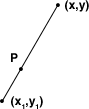Let $$(x,y)$$ be an arbitrary point in $$\mathbb R^2$$ and $$(x_1,y_1)$$ one of the vertices. We want to find a point $$P$$ that is at a fraction $$r$$ of the distance between $$(x_1,y_1)$$ and $$(x,y)$$. To find the x coordinate of $$P$$ we take $$x-x_1$$ to get the distance between $$(x,y)$$ and $$(x_1,y_1)$$ in the x axis and then multiply that by $$r$$ to get just the fraction of the distance. Then we just add $$x_1$$ to get the distace at that fraction starting from $$x_1$$. Putting all of this together, the x coordinate of $$P$$ is $$x_1 + r(x - x_1)$$. Notice that if $$x_1 > x_2$$ then $$r(x-x_1)$$ will be negative, and when added to $$x_1$$ we still get an x coordinate for $$P$$ which lies between $$x$$ and $$x_1$$. To get the y coordinate of $$P$$ we just follow exactly the same reasoning but with the y coordinates of the points.

Iterated functions systems are more general than what we have studied here and can produce many more fractals. An example of this is Barnsley's Fern which is depicted in Figure 5. For more information about this and other IFS check out Barnsley's book Fractasl Everywhere.

### Spicing Thigs Up

Now that we know how the chaos game works, we can start playing around with it. First let's add some color. The most obvious way to add color to the chaos game is to choose a color for each one of the mappings, and draw the point with the color of the mapping that was chosen for that particular point. We can take it a few steps further though, if we use the color of the mapping that was used for the last point drawn instead of the one picked for the current iteration, then we would not only be mapping the position of the points, but also the color the points would have had if we were using the previous coloring method. Try to figure out the effect this will have by yourself, if you want a hint, I suggest you take a look at Figure 4 above. Or if you're just here for the pretty pictures then take a look at Figure 6 which shows the Sierpinski triangle drawn using the color of the mapping picked anywhere between 0 and 6 points ago.

Figure 6. Chaos game coloring. Click here to show coloring using the color of the function picked: 0, 1, 2, 3, 4, 5, or 6 points ago.

Next, we can try playing around with the fraction. However most of the time the results of this will not be of much interest. For example, Figure 7 shows the chaos game with an equilateral triangle using the fractions $$\frac{2}{5}$$ (0.4) and $$\frac{3}{5}$$ (0.6).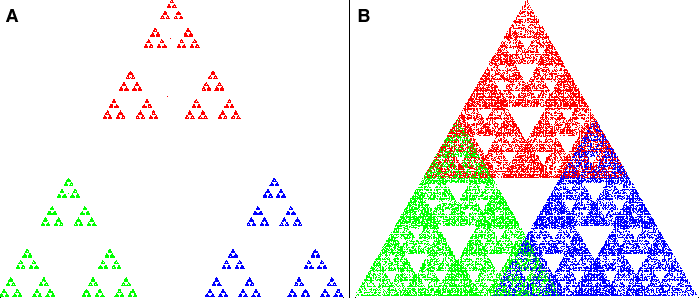Figure 7. A - Chaos game with $$n=3$$ and $$r=\frac{2}{5}$$ after 50,000 iterations. B - Chaos game with $$n=3$$ and $$r=\frac{3}{5}$$ after 50,000 iterations. Click to enlarge.

We can also try changing the the number of vertices and their positions. With three vertices changing the poistion of the vertices will not make much of a diference, we'll just get the Sierpinski triangle every time. Adding more vertices spices up the situation though. Take a look at Figure 8.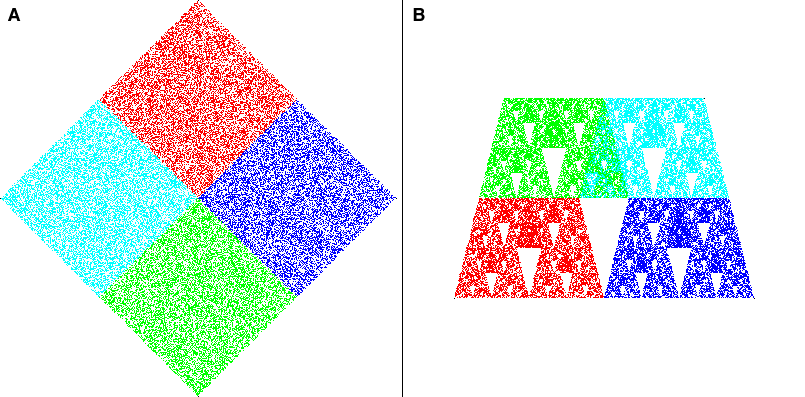Figure 8. A - Chaos game with $$n=4$$ and $$r=\frac{1}{2}$$ after 50,000 iterations. B - Chaos game with $$n=4$$ and $$r=\frac{1}{2}$$ after 50,000 iterations. Click to enlarge.

Figure 8-A is a bit more interesting than it might seem. The length of the sides of each of the squares mapped here has is exactly half the length of the sides of the big square. However, since four of these smaller squares fit exactly inside the big square we simply end up with a big square where every point has the same probability of being drawn. Figure 8-B shows the result of using a trapezoid as the polygon Notice that no matter which fraction we chose, the mappings will either overlap or leave some space between the small copies. For something a little bit more interesting, Figure 9 shows the Sierpinski carpet generated using the chaos game. Notice that here $$n=8$$ (the positions of the vertices are marked in black) and $$r=\frac{1}{3}$$.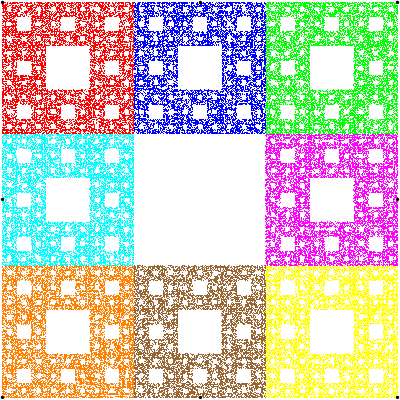Figure 9. Sierpinski carpet using the chaos game with $$n=8$$ and $$r=\frac{1}{3}$$ after 100,000 iterations.

So far it seems like tweaking the paramters of the chaos game at random is not going to produce nice images most of the time, so how can we get all the cool looking fractals? A natural idea is to try to copy what the Sierpinski triangle does with other regular polygons. Since we've settled on using regular polygons then we just need to figure out what the right fraction is. Our goal is to fit exactly $$n$$ shrinked copies of the polygon inside itself witout any overlap and the least empty space possible. We will call the fraction used to achive this the Sierpinski fraction and the fractals generated as a result Sierpinski n-gons.

In the next section we will se how to figure out the Sierpinski fraction for any regular polygon. It will get a bit technical so feel free to skip over to the last few paragraphs if you're not interested in the technical details. You can take a look at figures 12 and 14 if you just want to check out the results.

### The Sierpinski fraction

Consider an $$n$$-sided regular polygon with sides of length 1. We want to shrink that polygon so that $$n$$ copies of the shrunk polygon fit inside, one in each vertex. Let $$x$$ be length of the side of the shrunk copies and $$y$$ the distance between the vertex of the shrunk polygon after which the shrunk polygon's side and the original polygon's side no longer lie in the same line and the point where the side of the original polygon intersects with a line perpendicular to the side that passes through the closest vertex shared by two of the shrunk versions of the polygon. Figure 10 shows an illustration of this in a hexagon to make it easier to understand.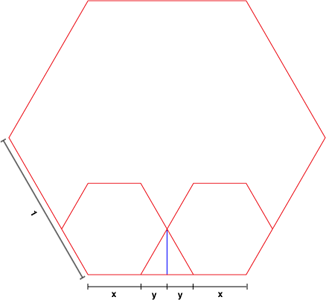Figure 10. $$x$$ and $$y$$ in a hexagon.

From the definitions of $$x$$ and $$y$$ we have that $$2x + 2y = 1$$ Also, since the length of the side of the original polygon is 1, then $$x$$ is the fraction by which we need to shrink the original polygon's side to get the side of the small polygon. This means that $$x$$ is actually the Sierpinski fraction for the polygon, so now we just need to get rid of $$y$$ anf solve for $$x$$.

To get rid of $$y$$ we will write it in terms of $$x$$. The first thing we should notice is that $$y$$ is the distance in the x axis between the polygon's vertex on the right side of $$x$$ and the vertex furthest to the right. Let's start by taking a look at what happens when $$n = 6$$. Figure 11 shows how $$y$$ looks like in this case.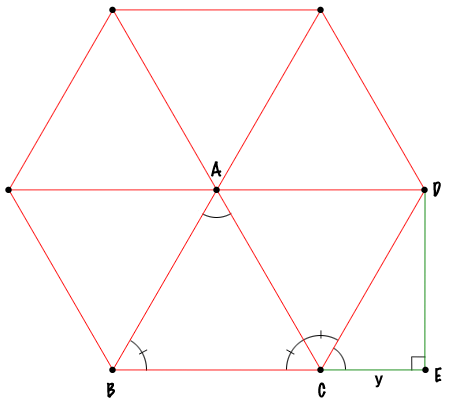Figure 11. $$y$$ and its relationship to the polygon's angles in a hexagon.

In Figure 11, notice that the segment $$CE$$ is precisely $$y$$. Also notice that segment $$DE$$ is perpendicular to $$CE$$ because of the way we defined $$y$$. Then, since $$\triangle CDE$$ is a right triangle and $$CD$$ is a side of the polygon $$y = x \cdot \cos(\angle DCE)$$. So if we had the value of $$\angle DCE$$ then we would be done. We know that we can split any regular $$n$$-gon into $$n$$ isosceles triangles. We also know that $$\angle BAC = \frac{2\pi}{n}$$ because all of the triangles that make up the regular polygon are congruent and the "top" angles in each of triangles added together must be equal to $$2 \pi$$ since they make a full turn. We also know that $$\angle ABC = \angle ACB$$ because the triangle is isosceles. And again, because all of the triangles that make up the polygon are congruent, then $$\angle ABC = \angle ACD$$. On the other hand, the sum of angles of a triangle is equal to $$\pi$$, so $$\angle BAC + \angle ABC + \angle ACB = \pi$$. But $$\angle ACB + \angle ACD + \angle DCE = \pi$$ because $$B$$, $$C$$ and $$E$$ are collinear by definition of $$E$$. Substiting, we get: $$\angle BAC + \angle ABC + \angle ACB = \angle ACB + \angle ACD + \angle DCE$$

but $$\angle ABC = \angle ACD$$, so substituting again: $$\angle BAC + \angle ABC + \angle ACB = \angle ACB + \angle ABC + \angle DCE$$

canceling $$\angle ABC + \angle ACB$$ on both sides we get: $$\angle BAC = \angle DCE$$, but $$\angle BAC = \frac{2\pi}{n}$$, so $$\angle DCE = \frac{2\pi}{n}$$.

Then, for the hexagon we have that $$y = x \cdot \cos(\angle DCE) = x \cdot cos(\frac{2\pi}{6}) = \frac{x}{2}$$ and since $$2x + 2y = 1$$, then, substituting yields: $$1 = 2x + 2(\frac{x}{2}) = 2x + x = 3x$$ so $$x = \frac{1}{3}$$. Which means that the Sierpinski fraction for the hexagon is $$\frac{1}{3}$$. Figure 12 shows how the chaos game looks like in a regular hexagon with $$r=\frac{1}{3}$$ .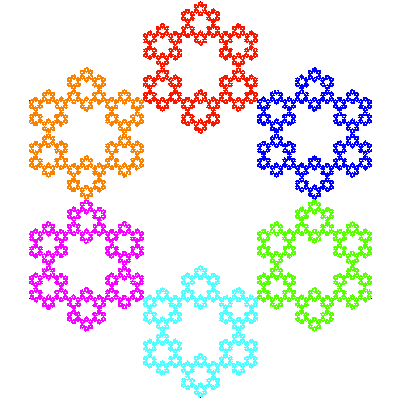Figure 12. Chaos game with $$n=6$$ and $$r=\frac{1}{3}$$ after 100,000 iterations.

What about other polygons? Unfortunately, calulating $$y$$ in other polygons is not so simple. To illustrate this, Figure 13 shows how $$y$$ looks like in a regular polygon with fourteen sides.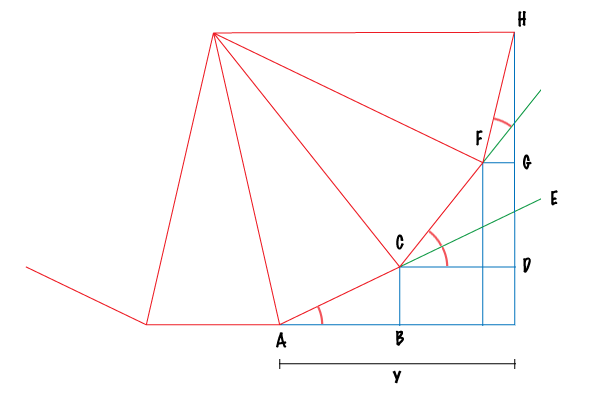Figure 13

In the general case, our approach will be to try to use the same reasoning we used with the hexagon to put $$y$$ in terms of a sum of cosines multiplied by $$x$$. There are two parts to this problem: first, we need to figure out the angles between each of the sides and and a horizontal line, then we need to figure out how many sides are located before the vertex furthest to the right. For the first part we can use what we did for the hexagon to see that $$\angle BAC = \frac{2 \pi}{n}$$. Since $$CD$$ is parallel to $$AB$$, then $$\angle BAC = \angle DCE$$ because they are corresponding angles betwen parallel lines. Also, by the same reasoning we used for $$\angle BAC$$, we get that $$\angle ECF = \frac{2 \pi}{n}$$. Lastly,

$$\angle DCF = \angle DCE + \angle ECF = \frac{2 \pi}{n} + \frac{2 \pi}{n} = 2 \left( \frac{2 \pi}{n} \right)$$

Using the same method we get that $$\angle GFH = 3 \left( \frac{2 \pi}{n} \right)$$. In general terms, each angle is the previous angle plus $$\frac{2\pi}{n}$$, or put another way, the $$i$$th angle is $$i \left( \frac{2\pi}{n}\right)$$.

Now we only need to figure out how many of these angles are before the vertex furthest to the right. To do this, we notice that a vertex will be further to the right than the previous vertex if and only if the angle between the side determined by the two vertices and a horizontal line is less than $$\frac{\pi}{2}$$. Since we know that these angles are precicely $$i \left(\frac{2 \pi}{n}\right)$$ then we just need to figure out for which values of $$i$$ the inequality

$$i \left(\frac{2 \pi}{n}\right) < \frac{\pi}{2}$$

holds true. Multiplying both sides by $$\frac{n}{2 \pi}$$ (which is positive since $$n>0$$) we get:

$$i < \frac{\pi}{2} \left( \frac{n}{2 \pi} \right) = \frac{n \pi}{4 \pi} = \frac{n}{4}$$

Since $$i$$ is an integer then $$i < \frac{n}{4}$$ if and only if $$i < \lfloor\frac{n}{4}\rfloor$$ (where $$\lfloor \cdot \rfloor$$ denotes the floor function), using this we can bring everythind together to get

$$y = \sum_{i = 1}^{\lfloor n/4 \rfloor} x \cdot \cos\left(i\left(\frac{2\pi}{n}\right)\right) = x \sum_{i = 1}^{\lfloor n/4 \rfloor} \cos\left(\frac{2\pi i}{n}\right)$$

And since $$2x + 2y = 1$$ then

\begin{align} 2x + 2\left(x \sum_{i = 1}^{\lfloor n/4 \rfloor} \cos\left(\frac{2\pi i}{n}\right)\right) &= 1 \\ 2x \left(1 + \sum_{i = 1}^{\lfloor n/4 \rfloor} \cos\left(\frac{2\pi i}{n}\right)\right) &= 1\\ x &= \frac{1}{2 \left(1 + \sum_{i = 1}^{\lfloor n/4 \rfloor} \cos\left(\frac{2\pi i}{n}\right)\right)} \end{align}

Recalling that $$x$$ was the value for the Sierpinski fraction, we are done. ■

Notice that even though we used the labels in our drawings above, the arguments we used hold true for any regular polygon and not just the ones we drew.

Now that we have figured out the Sierpinski fraction for any regular polygon, we can use it to draw some fractals using the chaos game. Figure 14 shows the chaos game using the Sierpinski fraction for $$n=5,7,8,9,10$$.

Figure 14. The Sierpinski fraction. Click here to show 100,000 iterations of the chaos game with the Sierpinski fraction for 5, 7, 8, 9, or 10 vertices.

As you can see for yourself, as the number of vertices goes up the fractals created using this method start to look like each other more and more. Also notices that for $$n=4$$ the Sierpinski fraction is $$\frac{1}{2}$$ so the so called "Sierpinski square" is actually the square in Figure 8-A. Finally, as Figure 8-B shows there are some polygons that don't have a Sierpinski fraction so we can't generalize it to all polygons.

You can play with the chaos game yourself using Chaotica for either mac or iPhone. Both applications were developed by me and the mac version was used for all the figures in this article.

Finally, if you enjoyed this article please consider donating by clicking the button below so that I can keep writing stuff like this. Any donation (no matter how big or small) is greatly appreciated!